# Superlinear gradient system with a parameter

## Abstract

In this paper, we study the multiplicity of nontrivial solutions for a superlinear gradient system with saddle structure at the origin. We make use of a combination of bifurcation theory, topological linking and Morse theory.

MSC:35J10, 35J65, 58E05.

## 1 Introduction

In this paper, we study the existence of multiple solutions to the gradient system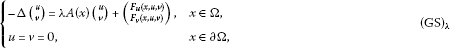where $\mathrm{\Omega }\subset {\mathbb{R}}^{N}$ is a bounded open domain with a smooth boundary Ω and $N⩾3$, λ is a real parameter and $A\in {\mathcal{M}}_{2}\left(\mathrm{\Omega }\right)$ is fixed. ${\mathcal{M}}_{2}\left(\mathrm{\Omega }\right)$ is the set of all continuous, cooperative and symmetric matrix functions on ${\mathbb{R}}^{2}$. A matrix function $A\in {\mathcal{M}}_{2}\left(\mathrm{\Omega }\right)$ takes the form

$A\left(x\right)=\left(\begin{array}{cc}a\left(x\right)& b\left(x\right)\\ b\left(x\right)& c\left(x\right)\end{array}\right)$

with the functions $a,b,c\in C\left(\overline{\mathrm{\Omega }},\mathbb{R}\right)$ satisfying the conditions that $b\left(x\right)⩾0$ for all $x\in \overline{\mathrm{\Omega }}$, which means A is cooperative and that ${max}_{x\in \overline{\mathrm{\Omega }}}\left\{a,c\right\}>0$.

We impose the following assumptions on the function F:

(${F}_{1}$) $F\in {C}^{2}\left(\mathrm{\Omega }×{\mathbb{R}}^{2},\mathbb{R}\right)$.

(${F}_{2}$) $F\left(x,0\right)=0$, $\mathrm{\nabla }F\left(x,0\right)=0$, ${F}_{z}^{″}\left(x,0\right)=0$ for $x\in \mathrm{\Omega }$.

(${F}_{3}$) There is $C>0$ and $2 such that

(${F}_{4}$) There is $\mu >2$, $M>0$ such that

$0<\mu F\left(x,z\right)⩽\left(\mathrm{\nabla }F\left(x,z\right),z\right)$

for all $x\in \mathrm{\Omega }$, $|z|⩾M$.

(${F}_{5}$) ${F}_{z}^{″}\left(x,z\right)>0$ for $|z|>0$ small and $x\in \mathrm{\Omega }$.

(${F}_{6}$) ${F}_{z}^{″}\left(x,z\right)<0$ for $|z|>0$ small and $x\in \mathrm{\Omega }$.

Here and in the sequel, 0 is used to denote the origin in various spaces, $|\cdot |$ and $\left(\cdot ,\cdot \right)$ denote the norm and the inner product in ${\mathbb{R}}^{2}$, Bz denotes the matrix product in ${\mathbb{R}}^{2}$ for a $2×2$ matrix B and $z=\left(u,v\right)\in {\mathbb{R}}^{2}$. For two symmetric matrices B and C in ${\mathbb{R}}^{2}$, $B>C$ means that $B-C$ is positive definite.

Let E be the Hilbert space ${H}_{0}^{1}\left(\mathrm{\Omega }\right)×{H}_{0}^{1}\left(\mathrm{\Omega }\right)$ endowed with the inner product

$〈z,w〉=〈\left(u,v\right),\left(\varphi ,\psi \right)〉={\int }_{\mathrm{\Omega }}\left(\mathrm{\nabla }u\mathrm{\nabla }\varphi +\mathrm{\nabla }v\mathrm{\nabla }\psi \right)\phantom{\rule{0.2em}{0ex}}dx,\phantom{\rule{1em}{0ex}}z=\left(u,v\right),w=\left(\varphi ,\psi \right)\in E$

and the associated norm

${\parallel z\parallel }^{2}={\int }_{\mathrm{\Omega }}{|\mathrm{\nabla }z|}^{2}\phantom{\rule{0.2em}{0ex}}dx={\int }_{\mathrm{\Omega }}{|\mathrm{\nabla }u|}^{2}+{|\mathrm{\nabla }v|}^{2}\phantom{\rule{0.2em}{0ex}}dx,\phantom{\rule{1em}{0ex}}z=\left(u,v\right)\in E.$

By the compact Sobolev embedding $E↪{L}^{p}\left(\mathrm{\Omega }\right)×{L}^{p}\left(\mathrm{\Omega }\right)$ for $p\in \left[2,{2}^{\ast }\right)$, under the assumptions (${F}_{1}$) and (${F}_{3}$), the functional

$\mathrm{\Phi }\left(z\right)=\frac{1}{2}{\int }_{\mathrm{\Omega }}{|\mathrm{\nabla }z|}^{2}-\lambda \left(A\left(x\right)z,z\right)\phantom{\rule{0.2em}{0ex}}dx-{\int }_{\mathrm{\Omega }}F\left(x,z\right)\phantom{\rule{0.2em}{0ex}}dx,\phantom{\rule{1em}{0ex}}z=\left(u,v\right)\in E$
(1.1)

is well defined and is of class ${C}^{2}$ (see ) with derivatives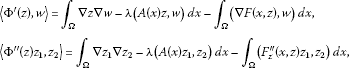for $z=\left(u,v\right)$, $w=\left(\varphi ,\psi \right)$, ${z}_{1}=\left({u}_{1},{v}_{1}\right)$, ${z}_{2}=\left({u}_{2},{v}_{2}\right)\in E$. Therefore, the solutions to (GS) λ are exactly critical points of Φ in E.

By (${F}_{2}$) the system (GS) λ admits a trivial solution $z=0$ for any fixed parameter $\lambda \in \mathbb{R}$. We are interested in finding nontrivial solutions to (GS) λ . The existence of nontrivial solutions of (GS) λ depends on the behaviors of F near zero and infinity. The purpose of this paper is to find multiple nontrivial solutions to (GS) λ with superlinear term when the trivial solution $z=0$ acts as a local saddle point of the energy functional Φ in the sense that the parameter λ is close to a higher eigenvalue of the linear gradient system with the given weight matrix A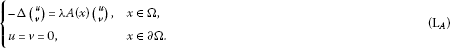It is known (see [2, 3]) that for a given matrix $A\in {\mathcal{M}}_{2}\left(\mathrm{\Omega }\right)$, (${\mathrm{L}}_{A}$) admits a sequence of distinct eigenvalues of finite multiplicity

$0<{\lambda }_{1}^{A}<{\lambda }_{2}^{A}<\cdots <{\lambda }_{k}^{A}<\cdots$

such that ${\lambda }_{k}^{A}\to \mathrm{\infty }$ as $k\to \mathrm{\infty }$.

Denote by ${F}^{-}$ the negative part of F, i.e., ${F}^{-}\left(x,z\right)=max\left\{-F\left(x,z\right),0\right\}$.

We will prove the following theorems.

Theorem 1.1 Assume (${F}_{1}$)-(${F}_{4}$), (${F}_{5}$) and let $k⩾1$ be fixed. Then there is $\delta >0$ such that when ${sup}_{\left(x,z\right)\in \mathrm{\Omega }×{\mathbb{R}}^{2}}{F}^{-}\left(x,z\right)⩽\delta$, for all $\lambda \in \left({\lambda }_{k+1}^{A}-\delta ,{\lambda }_{k+1}^{A}\right)$, (GS) λ has at least three nontrivial solutions in E.

Theorem 1.2 Assume (${F}_{1}$)-(${F}_{4}$), (${F}_{6}$) and let $k⩾1$ be fixed. Then there is $\delta >0$ such that when ${sup}_{\left(x,z\right)\in \mathrm{\Omega }×{\mathbb{R}}^{2}}{F}^{-}\left(x,z\right)⩽\delta$, for all $\lambda \in \left({\lambda }_{k+1}^{A},{\lambda }_{k+1}^{A}+\delta \right)$, (GS) λ has at least three nontrivial solutions in E.

Theorem 1.3 Assume (${F}_{1}$)-(${F}_{4}$) and $F⩽0$ for $x\in \mathrm{\Omega }$, $z\in {\mathbb{R}}^{2}$ with $|z|$ small. Then there is $\delta >0$ such that when ${sup}_{\left(x,z\right)\in \mathrm{\Omega }×{\mathbb{R}}^{2}}{F}^{-}\left(x,z\right)⩽\delta$, for all $\lambda \in \left({\lambda }_{k+1}^{A}-\delta ,{\lambda }_{k+1}^{A}\right]$, (GS) λ has at least two nontrivial solutions in E.

We give some comments and comparisons. The superlinear problems have been studied extensively via variational methods since the pioneering work of Ambrosetti and Rabinowitz . Most known results on elliptic superlinear problems are contributed to a single equation with Dirichlet boundary data. Let us mention some historical progress on a single equation. When the trivial solution 0 acted as a local minimizer of the energy functional, one positive solution and one negative solution were obtained by using the mountain-pass theorem in  and the cut-off techniques; and a third solution was constructed in a famous paper of Wang  by using a two dimensional linking method and a Morse theoretic approach. When the trivial solution 0 acted as a local saddle point of the energy functional, the existence of one nontrivial solution was obtained by applying a critical point theorem, which is now well known as the generalized mountain-pass theorem, built by Rabinowitz in  under a global sign condition (see ). Some extensions were done in [7, 8]via local linking. More recently, in the work of Rabinowitz, Su and Wang , multiple solutions have been obtained by combining bifurcation methods, Morse theory and homological linking when 0 is a saddle point in the sense that the parameter λ is very close to a higher eigenvalue of the related linear operator.

In the current paper, we build multiplicity results for superlinear gradient systems by applying the ideas constructed in . These results are new since, to the best of our knowledge, no multiplicity results for gradient systems have appeared in the literature for the case that $z=0$ is a saddle point of Φ.

We give some explanations regarding the conditions and conclusions. The assumptions (${F}_{1}$)-(${F}_{4}$) are standard in the study of superlinear problems. (${F}_{5}$) and (${F}_{6}$) are used for bifurcation analysis. It sees that (${F}_{5}$) implies that F is positive near zero, while (${F}_{6}$) implies that F must be negative near zero. The local properties of F near zero are necessary for constructing homological linking. When $F⩾0$, for any parameter λ in a bounded interval, say in $\left[{\lambda }_{k}^{A},{\lambda }_{k+1}^{A}\right)$, one can use the same arguments as in  to construct linking starting from ${\lambda }_{k+1}^{A}$. In our theorems, we do not require the global sign condition $F⩾0$. When the parameter λ is close to the eigenvalue ${\lambda }_{k+1}^{A}$, the homological linking will be constructed starting from ${\lambda }_{k+2}^{A}$ and this linking is different from the one in . This reveals the fact that when λ is close to ${\lambda }_{k+1}^{A}$ from the right-hand side, the linking starting from ${\lambda }_{k+2}^{A}$ can still be constructed even if F is negative somewhere. The conditions similar to (${F}_{5}$) and (${F}_{6}$) were first introduced in  where multiple periodic solutions for the second-order Hamiltonian systems were studied via the ideas in . Since we treat a different problem in the current paper, we need to present the detailed discussions although some arguments may be similar to those in [9, 10].

The paper is organized as follows. In Section 2, we collect some basic abstract tools. In Section 3, we get solutions by linking arguments and give partial estimates of homological information. In Section 4, we get solutions by bifurcation theorem and give the estimates of the Morse index. The final proofs of Theorems 1.1-1.3 are given in Section 5.

## 2 Preliminary

In this section, we give some preliminaries that will be used to prove the main results of the paper. We first collect some basic results on the Morse theory for a ${C}^{2}$ functional defined on a Hilbert space.

Let E be a Hilbert space and $\mathrm{\Phi }\in {C}^{2}\left(E,\mathbb{R}\right)$. Denote $\mathcal{K}=\left\{z\in E|{\mathrm{\Phi }}^{\prime }\left(z\right)=0\right\}$, ${\mathrm{\Phi }}^{c}=\left\{z\in E|\mathrm{\Phi }\left(z\right)⩽c\right\}$, ${\mathcal{K}}_{c}=\left\{z\in \mathcal{K}|\mathrm{\Phi }\left(z\right)=c\right\}$ for $c\in \mathbb{R}$. We say that Φ satisfies the (PS) c condition at the level $c\in \mathbb{R}$ if any sequence $\left\{{z}_{n}\right\}\subset E$ satisfying $\mathrm{\Phi }\left({z}_{n}\right)\to c$, ${\mathrm{\Phi }}^{\prime }\left({z}_{n}\right)\to 0$ as $n\to \mathrm{\infty }$, has a convergent subsequence. Φ satisfies (PS) if Φ satisfies (PS) c at any $c\in \mathbb{R}$.

We assume that Φ satisfies (PS) and $\mathrm{#}\mathcal{K}<\mathrm{\infty }$. Let ${z}_{0}\in \mathcal{K}$ with $\mathrm{\Phi }\left({z}_{0}\right)=c\in \mathbb{R}$ and U be a neighborhood of ${z}_{0}$ such that $U\cap \mathcal{K}=\left\{{z}_{0}\right\}$. The group

${C}_{q}\left(\mathrm{\Phi },{z}_{0}\right):={H}_{q}\left({\mathrm{\Phi }}^{c}\cap U,{\mathrm{\Phi }}^{c}\cap U\setminus \left\{{z}_{0}\right\}\right),\phantom{\rule{1em}{0ex}}q\in \mathbb{Z}$

is called the q th critical group of Φ at ${z}_{0}$, where ${H}_{\ast }\left(A,B\right)$ denotes a singular relative homology group of the pair $\left(A,B\right)$ with coefficients field $\mathbb{F}$ (see [11, 12]).

Let $a. The group

${C}_{q}\left(\mathrm{\Phi },\mathrm{\infty }\right):={H}_{q}\left(E,{\mathrm{\Phi }}^{a}\right),\phantom{\rule{1em}{0ex}}q\in \mathbb{Z}$

is called the q th critical group of Φ at infinity (see ).

We call ${M}_{q}:={\sum }_{z\in \mathcal{K}}dim{C}_{q}\left(\mathrm{\Phi },z\right)$ the q th Morse-type numbers of the pair $\left(E,{\mathrm{\Phi }}^{a}\right)$ and ${\beta }_{q}:=dim{C}_{q}\left(\mathrm{\Phi },\mathrm{\infty }\right)$ the Betti numbers of the pair $\left(E,{\mathrm{\Phi }}^{a}\right)$. The core of the Morse theory [11, 12] is the following relations between ${M}_{q}$ and ${\beta }_{q}$: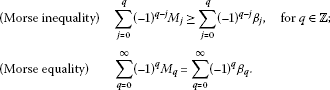If $\mathcal{K}=\mathrm{\varnothing }$, then ${\beta }_{q}=0$ for all q. Since ${M}_{q}⩾{\beta }_{q}$ for each $q\in \mathbb{Z}$, it follows that if ${\beta }_{{q}_{\ast }}\ne 0$ for some ${q}_{\ast }\in \mathbb{Z}$, then Φ must have a critical point ${z}_{\ast }$ with ${C}_{{q}_{\ast }}\left(\mathrm{\Phi },{z}_{\ast }\right)\ncong 0$. If $\mathcal{K}=\left\{{z}_{\ast }\right\}$, then ${C}_{q}\left(\mathrm{\Phi },\mathrm{\infty }\right)\cong {C}_{q}\left(\mathrm{\Phi },{z}_{\ast }\right)$ for all q. Thus, if ${C}_{q}\left(\mathrm{\Phi },\mathrm{\infty }\right)\ncong {C}_{q}\left(\mathrm{\Phi },{z}_{\ast }\right)$ for some q, then Φ must have a new critical point. One can use critical groups to distinguish critical points obtained by other methods and use the Morse equality to find new critical points.

For the critical groups of Φ at an isolated critical point, we have the following basic facts (see [11, 12]).

Proposition 2.1 Assume that z is an isolated critical point of $\mathrm{\Phi }\in {C}^{2}\left(E,\mathbb{R}\right)$ with a finite Morse index $m\left(z\right)$ and nullity $n\left(z\right)$. Then

1. (1)

${C}_{q}\left(\mathrm{\Phi },z\right)\cong {\delta }_{q,m\left(z\right)}\mathbb{F}$ if $n\left(z\right)=0$;

2. (2)

${C}_{q}\left(\mathrm{\Phi },z\right)\cong 0$ for $q\notin \left[m\left(z\right),m\left(z\right)+n\left(z\right)\right]$ (Gromoll-Meyer );

3. (3)

if ${C}_{m\left(z\right)}\left(\mathrm{\Phi },z\right)\ncong 0$ then ${C}_{q}\left(\mathrm{\Phi },z\right)\cong {\delta }_{q,m\left(z\right)}\mathbb{F}$;

4. (4)

if ${C}_{m\left(z\right)+n\left(z\right)}\left(\mathrm{\Phi },z\right)\ncong 0$ then ${C}_{q}\left(\mathrm{\Phi },z\right)\cong {\delta }_{q,m\left(z\right)+n\left(z\right)}\mathbb{F}$.

Proposition 2.2 ([15, 16])

Let 0 be an isolated critical point of $\mathrm{\Phi }\in {C}^{2}\left(E,\mathbb{R}\right)$ with a finite Morse index ${m}_{0}$ and nullity ${n}_{0}$. Assume that Φ has a local linking at 0 with respect to a direct sum decomposition $E={E}^{-}\oplus {E}^{+}$, $\kappa =dim{E}^{-}$, i.e., there exists $r>0$ small such that

$\mathrm{\Phi }\left(z\right)>0\phantom{\rule{1em}{0ex}}\mathit{\text{for}}\phantom{\rule{0.1em}{0ex}}z\in {E}^{+},0<\parallel z\parallel ⩽r,\phantom{\rule{2em}{0ex}}\mathrm{\Phi }\left(z\right)⩽0\phantom{\rule{1em}{0ex}}\mathit{\text{for}}\phantom{\rule{0.1em}{0ex}}z\in {E}^{-},\parallel z\parallel ⩽r.$

Then ${C}_{q}\left(\mathrm{\Phi },0\right)\cong {\delta }_{q,\kappa }\mathbb{Z}$ for either $\kappa ={m}_{0}$ or $\kappa ={m}_{0}+{n}_{0}$.

The concept of local linking was introduced in . In  a partial result was given for a ${C}^{1}$ functional. The above result was obtained in .

Now, we recall an abstract linking theorem which is from [1, 12, 15].

Proposition 2.3 ([1, 12, 15])

Let E be a real Banach space with $E=X\oplus Y$ and $\ell =dimX$ be finite. Suppose that $\mathrm{\Phi }\in {C}^{1}\left(E,\mathbb{R}\right)$ satisfies (PS) and

(${\mathrm{\Phi }}_{1}$) there exist $\rho >0$ and $\alpha >0$ such that

$\mathrm{\Phi }\left(z\right)⩾\alpha ,\phantom{\rule{1em}{0ex}}z\in {S}_{\rho }=Y\cap \partial {B}_{\rho },$
(2.1)

where ${B}_{\rho }=\left\{z\in E|\parallel z\parallel ⩽\rho \right\}$,

(${\mathrm{\Phi }}_{2}$) there exist $R>\rho >0$ and $e\in Y$ with $\parallel e\parallel =1$ such that

$\mathrm{\Phi }\left(z\right)<\alpha ,\phantom{\rule{1em}{0ex}}z\in \partial Q,$
(2.2)

where

$Q=\left\{z=y+se|\parallel z\parallel ⩽R,y\in X,0⩽s⩽R\right\}.$

Then Φ has a critical point ${z}_{\ast }$ with $\mathrm{\Phi }\left({z}_{\ast }\right)={c}_{\ast }⩾\alpha$ and

${C}_{\ell +1}\left(\mathrm{\Phi },{z}_{\ast }\right)\ne 0.$
(2.3)

We note here that under the framework of Proposition 2.3, ${S}_{\rho }$ and ∂Q homotopically link with respect to the direct sum decomposition $E=X\oplus Y$. ${S}_{\rho }$ and ∂Q are also homologically linked. The conclusion (2.3) follows from Theorems 1.1′ and 1.5 of Chapter II in . (See also .)

We finally collect some properties of the eigenvalue problem (${\mathrm{L}}_{A}$). Associated with a matrix $A\in {\mathcal{M}}_{2}\left(\mathrm{\Omega }\right)$, there is a compact self-adjoint operator ${T}_{A}:E\to E$ such that

$〈{T}_{A}z,w〉={\int }_{\mathrm{\Omega }}\left(A\left(x\right)z,w\right)\phantom{\rule{0.2em}{0ex}}dx,\phantom{\rule{1em}{0ex}}z,w\in E.$

The compactness of ${T}_{A}$ follows from the compact embedding $E↪{L}^{2}\left(\mathrm{\Omega }\right)×{L}^{2}\left(\mathrm{\Omega }\right)$. The operator ${T}_{A}$ possesses the property that ${\lambda }^{A}$ is an eigenvalue of (${\mathrm{L}}_{A}$) if and only if there is nonzero $z\in E$ such that

${\lambda }^{A}{T}_{A}z=z.$

(${\mathrm{L}}_{A}$) has the sequence of distinct eigenvalues

$0<{\lambda }_{1}^{A}<{\lambda }_{2}^{A}<\cdots <{\lambda }_{k}^{A}<\cdots \to \mathrm{\infty },$

and each eigenvalue ${\lambda }^{A}$ of (${\mathrm{L}}_{A}$) has a finite multiplicity. For $j\in \mathbb{N}$, denote

$E\left({\lambda }_{j}^{A}\right)=ker\left(-\mathrm{\Delta }-{\lambda }_{j}^{A}A\right),\phantom{\rule{2em}{0ex}}{E}_{j}=\underset{i=1}{\overset{j}{⨁}}E\left({\lambda }_{i}^{A}\right),\phantom{\rule{2em}{0ex}}{\ell }_{j}=dim{E}_{j}.$

Set

${Q}_{\lambda }\left(z\right)={\int }_{\mathrm{\Omega }}{|\mathrm{\nabla }z|}^{2}-\lambda \left(A\left(x\right)z,z\right)\phantom{\rule{0.2em}{0ex}}dx,\phantom{\rule{1em}{0ex}}z\in E.$

Then the following variational inequalities hold: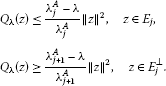We refer to [2, 3] for more properties related to the eigenvalue problem (${\mathrm{L}}_{A}$) and the operator ${T}_{A}$.

## 3 Solutions via homological linking

In this section, we give the existence a nontrivial solution of (GS) λ by applying homological linking arguments and then give some estimate of its Morse index. The following lemmas are needed.

Lemma 3.1 Assume that F satisfies (${F}_{1}$)-(${F}_{4}$), then for any fixed $\lambda \in \mathbb{R}$, the functional Φ satisfies the (PS) condition.

Proof By (${F}_{3}$) and the compact embedding $E↪{L}^{p}\left(\mathrm{\Omega }\right)×{L}^{p}\left(\mathrm{\Omega }\right)$ for $1⩽p<{2}^{\ast }$, it is enough to show that any sequence $\left\{{z}_{n}\right\}\subset E$ with

$|\mathrm{\Phi }\left({z}_{n}\right)|⩽C,\phantom{\rule{1em}{0ex}}n\in \mathbb{N},\phantom{\rule{2em}{0ex}}{\mathrm{\Phi }}^{\prime }\left({z}_{n}\right)\to 0,\phantom{\rule{1em}{0ex}}n\to \mathrm{\infty }$
(3.1)

is bounded in E. Here and below, we use C to denote various positive constants. We modify the arguments in . Choosing a positive number $\beta \in \left(1/\mu ,1/2\right)$ for n large, we have that

$C+\beta \parallel {z}_{n}\parallel ⩾\mathrm{\Phi }\left({z}_{n}\right)-\beta 〈{\mathrm{\Phi }}^{\prime }\left({z}_{n}\right),{z}_{n}〉.$

By (${F}_{4}$) we deduce that

(3.2)

Therefore,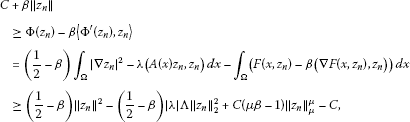where $\mathrm{\Lambda }={max}_{x\in \overline{\mathrm{\Omega }}}{\parallel A\left(x\right)\parallel }_{{\mathbb{R}}^{2}}$. By the Hölder inequality and the Young inequality, we get for any $ϵ>0$ that

${\parallel {z}_{n}\parallel }_{2}^{2}⩽{|\mathrm{\Omega }|}^{\frac{\mu -2}{\mu }}{\parallel {z}_{n}\parallel }_{\mu }^{2}⩽\frac{|\mathrm{\Omega }|\left(\mu -2\right)}{\mu }{ϵ}^{\frac{2}{2-\mu }}+\frac{2}{\mu }ϵ{\parallel {z}_{n}\parallel }_{\mu }^{\mu }.$
(3.3)

Thus, for a fixed $ϵ>0$ small enough, we have by (3.3) that

$C+\beta \parallel {z}_{n}\parallel ⩾\left(\frac{1}{2}-\beta \right){\parallel {z}_{n}\parallel }^{2}+\frac{1}{2}C\left(\mu \beta -1\right){\parallel {z}_{n}\parallel }_{\mu }^{\mu }.$

Therefore, $\left\{{z}_{n}\right\}$ is bounded in E. The proof is complete. □

Now, we construct a homological linking with respect to the direct sum decomposition of E for $k⩾1$:

$E={E}_{k+1}\oplus {E}_{k+1}^{\perp },\phantom{\rule{2em}{0ex}}dim{E}_{k+1}={\ell }_{k+1}.$

Take an eigenvector ${\varphi }_{k+2}$ corresponding to the eigenvalue ${\lambda }_{k+2}^{A}$ of (${\mathrm{L}}_{A}$) with $\parallel {\varphi }_{k+2}\parallel =1$. Set

${V}_{k+1}:={E}_{k+1}\oplus span\left\{{\varphi }_{k+2}\right\}.$

Lemma 3.2 Assume that F satisfies (${F}_{1}$)-(${F}_{4}$) and $k⩾1$. Then there exist constants $\rho >0$ small, $\alpha >0$ such that for all $\lambda ⩽{\lambda }_{\ast }:=\frac{{\lambda }_{k+2}^{A}+{\lambda }_{k+1}^{A}}{2}$, such that

$\mathrm{\Phi }\left(z\right)⩾\alpha ,\phantom{\rule{1em}{0ex}}\mathit{\text{for}}\phantom{\rule{0.1em}{0ex}}z\in {E}_{k+1}^{\mathrm{\perp }}\phantom{\rule{0.1em}{0ex}}\mathit{\text{with}}\phantom{\rule{0.1em}{0ex}}\parallel z\parallel =\rho .$
(3.4)

Proof By the conditions (${F}_{2}$) and (${F}_{3}$), for $ϵ>0$, there is ${C}_{ϵ}>0$ such that

$F\left(x,z\right)⩽\frac{1}{2{S}_{2}}ϵ{|z|}^{2}+{C}_{ϵ}{|z|}^{p},$

where ${S}_{2}$ is the constant for the embedding $E↪{L}^{2}\left(\mathrm{\Omega }\right)×{L}^{2}\left(\mathrm{\Omega }\right)$ such that ${|z|}_{2}^{2}⩽{S}_{2}{\parallel z\parallel }^{2}$ for $z\in E$. Since for $z\in {E}_{k+1}^{\mathrm{\perp }}$,

${Q}_{\lambda }\left(z\right)={\int }_{\mathrm{\Omega }}{|\mathrm{\nabla }z|}^{2}-\lambda \left(A\left(x\right)z,z\right)\phantom{\rule{0.2em}{0ex}}dx⩾\frac{{\lambda }_{k+2}^{A}-\lambda }{{\lambda }_{k+2}^{A}}{\parallel z\parallel }^{2},$

it follows that

$\begin{array}{rcl}\mathrm{\Phi }\left(z\right)& =& \frac{1}{2}{Q}_{\lambda }\left(z\right)-{\int }_{\mathrm{\Omega }}F\left(x,z\right)\phantom{\rule{0.2em}{0ex}}dx\\ ⩾& \frac{1}{2}\frac{{\lambda }_{k+2}^{A}-\lambda }{{\lambda }_{k+2}^{A}}{\parallel z\parallel }^{2}-\frac{1}{2{S}_{2}}ϵ{\int }_{\mathrm{\Omega }}{|z|}^{2}\phantom{\rule{0.2em}{0ex}}dx-{C}_{ϵ}{\int }_{\mathrm{\Omega }}{|z|}^{p}\phantom{\rule{0.2em}{0ex}}dx\\ ⩾& \frac{1}{2}\frac{{\lambda }_{k+2}^{A}-\lambda -ϵ}{{\lambda }_{k+2}^{A}}{\parallel z\parallel }^{2}-{\stackrel{˜}{C}}_{ϵ}{\parallel z\parallel }^{p}\\ =& \frac{1}{2}\eta \left(\lambda ,ϵ\right){\parallel z\parallel }^{2}-{\stackrel{˜}{C}}_{ϵ}{\parallel z\parallel }^{p},\end{array}$

where ${\stackrel{˜}{C}}_{ϵ}$ is independent of λ and

$\eta \left(\lambda ,ϵ\right)=\frac{{\lambda }_{k+2}^{A}-\lambda -ϵ}{{\lambda }_{k+2}^{A}}.$
(3.5)

Since $p>2$ and the function $g\left(r\right)=\frac{1}{2}\eta \left(\lambda ,ϵ\right){r}^{2}-{\stackrel{˜}{C}}_{ϵ}{r}^{p}$ achieves its maximum

${g}_{max}=\frac{p-2}{2p}{\left(p{\stackrel{˜}{C}}_{ϵ}\right)}^{\frac{2}{2-p}}\eta {\left(\lambda ,ϵ\right)}^{\frac{p}{p-2}}:=\alpha \left(\lambda ,ϵ\right)$
(3.6)

on $\left(0,\mathrm{\infty }\right)$ at

${\rho }_{\lambda ,ϵ}={\left(\frac{\eta \left(\lambda ,ϵ\right)}{p{\stackrel{˜}{C}}_{ϵ}}\right)}^{\frac{1}{p-2}},$
(3.7)

we see that

(3.8)

Since $\eta \left(\lambda ,ϵ\right)$ is a decreasing function with respect to λ for any fixed $ϵ>0$ small, (3.4) holds for

The constants α and ρ are independent of $\lambda ⩽{\lambda }_{\ast }$. The proof is complete. □

Lemma 3.3 Assume that F satisfies (${F}_{1}$), (${F}_{4}$) and $k⩾1$. Then there exist $R>0$, $\delta >0$ and $\sigma \in \mathbb{R}$, all independent of λ, such that when $\lambda \in \left({\lambda }_{k+1}^{A}-\delta ,{\lambda }_{k+1}^{A}+\delta \right)$ and ${sup}_{\left(x,z\right)\in \mathrm{\Omega }×{\mathbb{R}}^{2}}{F}^{-}\left(x,z\right)⩽\delta$,

$\mathrm{\Phi }\left(z\right)⩽\sigma <\alpha ,\phantom{\rule{1em}{0ex}}\mathit{\text{for}}\phantom{\rule{0.1em}{0ex}}z\in \partial Q,$
(3.9)

where

$Q=\left\{z\in {V}_{k+1}|\parallel z\parallel ⩽R,z=y+s{\varphi }_{k+2},y\in {E}_{k+1},s⩾0\right\}.$

Proof From (${F}_{4}$) we deduce (3.2) with a positive constant C independent of λ. For $z\in {V}_{k+1}$, write $z=y+w+\varphi$, $y\in {E}_{k}$, $w\in E\left({\lambda }_{k+1}^{A}\right)$, $\varphi \in span\left\{{\varphi }_{k+2}\right\}$. Assume that $\lambda \in \left({\lambda }_{k}^{A},{\lambda }_{k+2}^{A}\right)$, then

$\begin{array}{rcl}\mathrm{\Phi }\left(z\right)& =& \frac{1}{2}{Q}_{\lambda }\left(y\right)+\frac{1}{2}{Q}_{\lambda }\left(w\right)+\frac{1}{2}{Q}_{\lambda }\left(\varphi \right)-{\int }_{\mathrm{\Omega }}F\left(x,z\right)\phantom{\rule{0.2em}{0ex}}dx\\ ⩽& \frac{{\lambda }_{k}^{A}-\lambda }{2{\lambda }_{k}^{A}}{\parallel y\parallel }^{2}+\frac{{\lambda }_{k+1}^{A}-\lambda }{2{\lambda }_{k+1}^{A}}{\parallel w\parallel }^{2}+\frac{{\lambda }_{k+2}^{A}-\lambda }{2{\lambda }_{k+2}^{A}}{\parallel \varphi \parallel }^{2}-C{\parallel z\parallel }_{\mu }^{\mu }+C\\ ⩽& \frac{{\lambda }_{k+2}^{A}-{\lambda }_{k}^{A}}{2{\lambda }_{k+1}^{A}}{\parallel z\parallel }^{2}-C{\parallel z\parallel }_{\mu }^{\mu }+C.\end{array}$
(3.10)

Since $\mu >2$ and $dim{V}_{k+1}<\mathrm{\infty }$, (3.10) shows that there exists $R>0$ independent of λ such that

(3.11)

Now, fix such an $R>0$ that $R>\rho$ with ρ given in Lemma 3.2. For $y\in {E}_{k+1}$ with $\parallel y\parallel ⩽R$, we write $y=w+\varphi$, $w\in {E}_{k}$, $\varphi \in E\left({\lambda }_{k+1}^{A}\right)$. Set $\mathrm{\Gamma }:={sup}_{\left(x,z\right)\in \mathrm{\Omega }×{\mathbb{R}}^{2}}{F}^{-}\left(x,z\right)$. Then we have that

$\begin{array}{rcl}\mathrm{\Phi }\left(y\right)& =& \frac{1}{2}{Q}_{\lambda }\left(w\right)+\frac{1}{2}{Q}_{\lambda }\left(\varphi \right)-{\int }_{\mathrm{\Omega }}F\left(x,y\right)\phantom{\rule{0.2em}{0ex}}dx\\ ⩽& \frac{{\lambda }_{k}^{A}-\lambda }{2{\lambda }_{k}^{A}}{\parallel w\parallel }^{2}+\frac{{\lambda }_{k+1}^{A}-\lambda }{2{\lambda }_{k+1}^{A}}{\parallel \varphi \parallel }^{2}+{\int }_{\left\{x\in \mathrm{\Omega }:F⩽0\right\}}{F}^{-}\left(x,y\right)\phantom{\rule{0.2em}{0ex}}dx\\ ⩽& \frac{|{\lambda }_{k+1}^{A}-\lambda |}{2{\lambda }_{k+1}^{A}}{R}^{2}+|\mathrm{\Omega }|\mathrm{\Gamma }.\end{array}$
(3.12)

Since $\partial Q=\left\{z=y+s{\varphi }_{k+1}|\parallel z\parallel =R,y\in {E}_{k},s⩾0\right\}\cup \left\{y\in {E}_{k}|\parallel y\parallel ⩽R\right\}$, taking

$\delta ={\left(\frac{{R}^{2}}{{\lambda }_{k+1}^{A}}+2|\mathrm{\Omega }|\right)}^{-1}\alpha ,\phantom{\rule{2em}{0ex}}\sigma =\frac{\alpha }{2},$

then, when $\lambda \in \left({\lambda }_{k+1}^{A}-\delta ,{\lambda }_{k+1}^{A}+\delta \right)$ and $\mathrm{\Gamma }⩽\delta$,

The proof is complete. □

Now, we apply Proposition 2.3 to get the following existence result with partial homological information.

Theorem 3.4 Let F satisfy (${F}_{1}$)-(${F}_{4}$) and $k⩾1$. Then there is $\delta >0$ such that when $\mathrm{\Gamma }⩽\delta$, for each $\lambda \in \left({\lambda }_{k+1}^{A}-\delta ,{\lambda }_{k+1}^{A}+\delta \right)$, (GS) λ has one nontrivial solution ${z}^{\ast }$ with a critical group satisfying

${C}_{{\ell }_{k+1}+1}\left(\mathrm{\Phi },{z}^{\ast }\right)\ncong 0.$
(3.13)

Proof By Lemma 3.1, Φ satisfies (PS). By Lemmas 3.2 and 3.3, for each fixed $\lambda \in \left({\lambda }_{k+1}-\delta ,{\lambda }_{k+1}+\delta \right)$, Φ satisfies (${\mathrm{\Phi }}_{1}$) and (${\mathrm{\Phi }}_{2}$) in the sense that

$\left\{\begin{array}{cc}\left({\mathrm{\Phi }}_{1}\right):\mathrm{\Phi }\left(z\right)⩾\alpha ,\hfill & z\in {S}_{k+1}:={S}_{\rho }\cap {E}_{k+1};\hfill \\ \left({\mathrm{\Phi }}_{2}\right):\mathrm{\Phi }\left(y\right)⩽\sigma <\alpha ,\hfill & y\in \partial Q.\hfill \end{array}$
(3.14)

Since ${S}_{k+1}$ and ∂Q homotopically link with respect to the decomposition $E={E}_{k+1}\oplus {E}_{k+1}^{\perp }$, and $dim{V}_{k+1}={\ell }_{k+1}+1$, it follows from Proposition 2.3 that Φ has a critical point ${z}^{\ast }\in E$ with positive energy $\mathrm{\Phi }\left({z}^{\ast }\right)⩾\alpha >0$ and its critical group satisfying (3.13). The proof is complete. □

We give some remarks. The existence of one nontrivial solution in Theorem 3.4 is valid when F is of class ${C}^{1}$. From Lemma 3.2, one sees that the energy of the obtained solution is bounded away from 0 as λ is close to ${\lambda }_{k+1}^{A}$. A rough local sign condition on F is needed. If $F⩾0$, then for any fixed $\lambda \in \left[{\lambda }_{k}^{A},{\lambda }_{k+1}^{A}\right)$, a linking with respect to ${E}_{k}\oplus {E}_{k}^{\perp }$ can be constructed. Proposition 2.3 is applied again to get a nontrivial solution ${z}_{\ast }$ satisfying

${C}_{{\ell }_{k}+1}\left(\mathrm{\Phi },{z}_{\ast }\right)\ncong 0.$
(3.15)

Therefore, when a global sign condition $F⩾0$ is present, as λ is close to ${\lambda }_{k+1}^{A}$ from the left-hand side, two linkings can be constructed and two nontrivial solutions can be obtained. The question is how to distinguish ${z}^{\ast }$ from ${z}_{\ast }$. Theorem 3.4 includes the case that for λ close to ${\lambda }_{k+1}^{A}$ from the right-hand side, the linking with respect to ${E}_{k+1}\oplus {E}_{k+1}^{\perp }$ is constructed provided the negative values of F are small. This phenomenon was first observed in .

## 4 Solutions via bifurcation

In this section, we get two solutions for (GS) λ via bifurcation arguments . We first cite the bifurcation theorem in .

Proposition 4.1 (Theorem 11.35 in )

Let E be a Hilbert space and $\mathrm{\Psi }\in {C}^{2}\left(E,\mathbb{R}\right)$ with

$\mathrm{\nabla }\mathrm{\Psi }\left(u\right)=Lu+H\left(u\right),$

where $L\in \mathcal{L}\left(E,E\right)$ is symmetric and $H\left(u\right)=o\left(\parallel u\parallel \right)$ as $\parallel u\parallel \to 0$. Consider the equation

$Lu+H\left(u\right)=\lambda u.$
(4.1)

Let $\mu \in \sigma \left(L\right)$ be an isolated eigenvalue of finite multiplicity. Then either

1. (i)

$\left(\mu ,0\right)$ is not an isolated solution of (4.1) in $\left\{\mu \right\}×E$, or

2. (ii)

there is a one-sided neighborhood Λ of μ such that for all $\lambda \in \mathrm{\Lambda }\setminus \left\{\mu \right\}$, (4.1) has at least two distinct nontrivial solutions, or

3. (iii)

there is a neighborhood Λ of μ such that for all $\lambda \in \mathrm{\Lambda }\setminus \left\{\mu \right\}$, (4.1) has at least one nontrivial solution.

We apply Proposition 4.1 to get two nontrivial solutions of (GS) λ for λ close to an eigenvalue of (${\mathrm{L}}_{A}$) and then give the estimates of the Morse index.

Theorem 4.2 Assume that F satisfies (${F}_{1}$)-(${F}_{3}$). Let $k⩾1$ be fixed. Then there exists $\delta >0$ such that (GS) λ has at least two nontrivial solutions for

1. (1)

every $\lambda \in \left({\lambda }_{k+1}^{A}-\delta ,{\lambda }_{k+1}^{A}\right)$ if (${F}_{5}$) holds;

2. (2)

every $\lambda \in \left({\lambda }_{k+1}^{A},{\lambda }_{k+1}^{A}+\delta \right)$ if (${F}_{6}$) holds.

Furthermore, the Morse index $m\left({z}_{\lambda }\right)$ and the nullity $n\left({z}_{\lambda }\right)$ of such a solution ${z}_{\lambda }$ satisfy

${\ell }_{k}⩽m\left({z}_{\lambda }\right)⩽m\left({z}_{\lambda }\right)+n\left({z}_{\lambda }\right)⩽{\ell }_{k+1},\phantom{\rule{1em}{0ex}}\mathit{\text{for}}\phantom{\rule{0.1em}{0ex}}0<|\lambda -{\lambda }_{k+1}^{A}|<\delta .$
(4.2)

Proof Under the assumptions (${F}_{1}$)-(${F}_{2}$), for each eigenvalue ${\lambda }_{j}^{A}$ of (${\mathrm{L}}_{A}$), $\left({\lambda }_{j}^{A},0\right)$ is a bifurcation point of (GS) λ (see ).

Let $\left(\lambda ,z\right)\in \mathbb{R}×E$ be a solution of (GS) λ near $\left({\lambda }_{k+1}^{A},0\right)$ which satisfies

$\left\{\begin{array}{cc}-\mathrm{\Delta }z=\lambda A\left(x\right)z+\mathrm{\nabla }F\left(x,z\right),\hfill & x\in \mathrm{\Omega },\hfill \\ z=\left(u,v\right)=0,\hfill & x\in \partial \mathrm{\Omega }.\hfill \end{array}$
(4.3)

By (${F}_{1}$) and (${F}_{2}$), we have

(4.4)

Let (${F}_{5}$) hold. By the elliptic regularity theory (see ), $\parallel z\parallel >0$ small implies ${\parallel z\parallel }_{C}>0$ small. Then by (${F}_{5}$), we have that

${F}_{z}^{″}\left(z,\eta z\left(x\right)\right)>0,\phantom{\rule{1em}{0ex}}x\in \mathrm{\Omega }.$
(4.5)

Now, consider the linear eigenvalue gradient system:

$\left\{\begin{array}{cc}-\mathrm{\Delta }y-{F}_{z}^{″}\left(x,\eta z\left(x\right)\right)y=\mu A\left(x\right)y,\hfill & x\in \mathrm{\Omega },\hfill \\ y=0,\hfill & x\in \partial \mathrm{\Omega }.\hfill \end{array}$
(4.6)

We denote the distinct eigenvalues of (4.6) by ${\mu }_{1}\left(z\right)<{\mu }_{2}\left(z\right)<\cdots <{\mu }_{i}\left(z\right)<\cdots$ as $z\ne 0$. By (${F}_{2}$), if we take $z=0$, then for each $i\in \mathbb{N}$, there is $j\in \mathbb{N}$ such that ${\mu }_{i}\left(0\right)={\lambda }_{j}^{A}$. By (4.5), the standard variational characterization of the eigenvalues of (4.6) shows that ${\mu }_{i}\left(z\right)$ is less than the corresponding j th ordered eigenvalue ${\lambda }_{j}^{A}$ of (${\mathrm{L}}_{A}$). Furthermore, ${\mu }_{i}\left(z\right)\to {\lambda }_{j}^{A}$ as $z\to 0$ in E. By (4.3) and (4.4), we see that z is a solution of (4.6) with eigenvalue λ. It must be that $\lambda <{\lambda }_{k+1}^{A}$ since λ is close to ${\lambda }_{k+1}^{A}$. Therefore, the case (ii) of Proposition 4.1 occurs under the given conditions. This proves the case (1). The existence for the case (2) is proved in the same way.

Now, we estimate the Morse indices for the solutions obtained above. Let ${z}_{\lambda }$ be a bifurcation solution of (GS) λ . Then

Applying the elliptic regularity theory, we have that

${\parallel {z}_{\lambda }\parallel }_{C}\to 0,\phantom{\rule{1em}{0ex}}\lambda \to {\lambda }_{k+1}^{A}.$
(4.7)

For each $y\in E$, we have

$〈{\mathrm{\Phi }}^{″}\left({z}_{\lambda }\right)y,y〉={Q}_{\lambda }\left(y\right)-{\int }_{\mathrm{\Omega }}\left({F}_{z}^{″}\left(x,{z}_{\lambda }\right)y,y\right)\phantom{\rule{0.2em}{0ex}}dx.$

Therefore, for $y\in {E}_{k}$,

$〈{\mathrm{\Phi }}^{″}\left({z}_{\lambda }\right)y,y〉⩽\frac{{\lambda }_{k}^{A}-\lambda }{{\lambda }_{k}^{A}}{\parallel y\parallel }^{2}+{\int }_{\mathrm{\Omega }}|\left({F}_{z}^{″}\left(x,{z}_{\lambda }\right)y,y\right)|\phantom{\rule{0.2em}{0ex}}dx,$

and for $\varphi \in {E}_{k+1}^{\mathrm{\perp }}$,

$〈{\mathrm{\Phi }}^{″}\left({z}_{\lambda }\right)\varphi ,\varphi 〉⩾\frac{{\lambda }_{k+2}^{A}-\lambda }{{\lambda }_{k+2}^{A}}{\parallel \varphi \parallel }^{2}-{\int }_{\mathrm{\Omega }}|\left({F}_{z}^{″}\left(x,{z}_{\lambda }\right)\varphi ,\varphi \right)|\phantom{\rule{0.2em}{0ex}}dx.$

By (${F}_{2}$) and (4.7), there exists $\delta >0$ such that when $0<|\lambda -{\lambda }_{k+1}^{A}|<\delta$,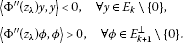Therefore, the Morse index $m\left({z}_{\lambda }\right)$ and the nullity $n\left({z}_{\lambda }\right)$ of ${z}_{\lambda }$ satisfy (4.2). The proof is complete. □

## 5 Proofs of main theorems

In this section, we give the proof of main theorems in this paper. We first compute the critical groups of Φ at both infinity and zero.

Lemma 5.1 (see )

Let F satisfy (${F}_{1}$)-(${F}_{4}$), then for any fixed $\lambda \in \mathbb{R}$,

${C}_{q}\left(\mathrm{\Phi },\mathrm{\infty }\right)\cong 0,\phantom{\rule{1em}{0ex}}\mathit{\text{for all}}\phantom{\rule{0.1em}{0ex}}q\in \mathbb{Z}.$
(5.1)

Proof The idea of the proof comes from the famous paper . We include a sketched proof in an abstract version. Given $\lambda \in \mathbb{R}$, denote ${B}_{1}=\left\{z\in E:\parallel z\parallel ⩽1\right\}$, ${S}_{1}=\partial {B}_{1}$. Modifying the arguments in , we get the following facts: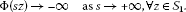(5.2)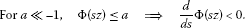(5.3)

The following arguments are from . As $\mathrm{\Phi }\left(0\right)=0$, it follows from (5.2) and (5.3) that for each $z\in {S}_{1}$, there is a unique $\tau \left(z\right)>0$ such that

$\mathrm{\Phi }\left(\tau \left(z\right)z\right)=a.$
(5.4)

By (5.4) and the implicit function theorem, we have that $\tau \in C\left({S}_{1},\mathbb{R}\right)$. Define

Then $\pi \in C\left(E\setminus \left\{0\right\},\mathbb{R}\right)$. Define a map $\varrho :\left[0,1\right]×E\setminus \left\{0\right\}\to E\setminus \left\{0\right\}$ by

$\varrho \left(t,z\right)=\left(1-t\right)z+t\pi \left(z\right)z.$
(5.5)

Clearly, ϱ is continuous, and for all $z\in E\setminus \left\{0\right\}$ with $\mathrm{\Phi }\left(z\right)>a$, by (5.4),

$\mathrm{\Phi }\left(\varrho \left(1,z\right)\right)=\mathrm{\Phi }\left(\pi \left({\parallel z\parallel }^{-1}z\right){\parallel z\parallel }^{-1}z\right)=a.$

Therefore,

and so ${\mathrm{\Phi }}^{a}$ is a strong deformation retract of $E\setminus \left\{0\right\}$. Hence,

${C}_{q}\left(\mathrm{\Phi },\mathrm{\infty }\right):={H}_{q}\left(E,{\mathrm{\Phi }}^{a}\right)\cong {H}_{q}\left(E,E\setminus \left\{0\right\}\right)\cong {H}_{q}\left({B}_{1},{S}_{1}\right)\cong 0,\phantom{\rule{1em}{0ex}}q\in \mathbb{Z}$

since ${S}_{1}$ is contractible, which follows from the fact that $dimE=\mathrm{\infty }$. □

Lemma 5.2 Let F satisfy (${F}_{1}$)-(${F}_{3}$).

1. (1)

For $\lambda \in \left({\lambda }_{k}^{A},{\lambda }_{k+1}^{A}\right)$, ${C}_{q}\left(\mathrm{\Phi },0\right)\cong {\delta }_{q,{\ell }_{k}}\mathbb{F}$.

2. (2)

For $\lambda \in \left({\lambda }_{k+1}^{A},{\lambda }_{k+2}^{A}\right)$, ${C}_{q}\left(\mathrm{\Phi },0\right)\cong {\delta }_{q,{\ell }_{k+1}}\mathbb{F}$.

3. (3)

For $\lambda ={\lambda }_{k+1}^{A}$, if $F\left(x,z\right)⩽0$ for $|z|$ small, then ${C}_{q}\left(\mathrm{\Phi },0\right)\cong {\delta }_{q,{\ell }_{k}}\mathbb{F}$.

4. (4)

For $\lambda ={\lambda }_{k+1}^{A}$, if $F\left(x,z\right)⩾0$ for $|z|$ small, then ${C}_{q}\left(\mathrm{\Phi },0\right)\cong {\delta }_{q,{\ell }_{k+1}}\mathbb{F}$.

Proof By (${F}_{2}$), we have

$〈{\mathrm{\Phi }}^{″}\left(0\right)y,y〉={Q}_{\lambda }\left(y\right)={\int }_{\mathrm{\Omega }}{|\mathrm{\nabla }y|}^{2}-\lambda \left(A\left(x\right)y,y\right)\phantom{\rule{0.2em}{0ex}}dx,\phantom{\rule{1em}{0ex}}y\in E.$
1. (1)

When $\lambda \in \left({\lambda }_{k}^{A},{\lambda }_{k+1}^{A}\right)$, $z=0$ is a nondegenerate critical point of Φ with the Morse index ${m}_{0}={\ell }_{k}$, thus ${C}_{q}\left(\mathrm{\Phi },0\right)\cong {\delta }_{q,{\ell }_{k}}\mathbb{F}$.

2. (2)

When $\lambda \in \left({\lambda }_{k+1}^{A},{\lambda }_{k+2}^{A}\right)$, $z=0$ is a nondegenerate critical point of Φ with the Morse index ${m}_{0}={\ell }_{k+1}$, thus ${C}_{q}\left(\mathrm{\Phi },0\right)\cong {\delta }_{q,{\ell }_{k+1}}\mathbb{F}$.

3. (3)

When $\lambda ={\lambda }_{k+1}^{A}$, $z=0$ is a degenerate critical point of Φ with the Morse index ${m}_{0}={\ell }_{k}$ and the nullity ${n}_{0}=dimE\left({\lambda }_{k+1}^{A}\right)$, ${m}_{0}+{n}_{0}={\ell }_{k+1}$.

Assume that $F\left(x,z\right)⩽0$ for $|z|⩽\sigma$ with $\sigma >0$ small. We will show that Φ has a local linking structure at $z=0$ with respect to $E={E}_{k}\oplus {E}_{k}^{\perp }$. If this has been done, then by Proposition 2.2, we have ${C}_{q}\left(\mathrm{\Phi },0\right)\cong {\delta }_{q,{\ell }_{k}}\mathbb{F}$.

Now, Φ can be written as

$\mathrm{\Phi }\left(z\right)=\frac{1}{2}{Q}_{{\lambda }_{k+1}^{A}}\left(z\right)-{\int }_{\mathrm{\Omega }}F\left(x,z\right)\phantom{\rule{0.2em}{0ex}}dx.$

By (${F}_{2}$) and (${F}_{3}$), for $ϵ>0$, there is ${C}_{ϵ}>0$ such that

$F\left(x,z\right)⩽\frac{1}{2}ϵ{|z|}^{2}+{C}_{ϵ}{|z|}^{p},\phantom{\rule{1em}{0ex}}z\in {\mathbb{R}}^{2},x\in \mathrm{\Omega }.$

Hence, for $z\in {E}_{k}$, we have that

$\mathrm{\Phi }\left(z\right)⩽\frac{{\lambda }_{k}^{A}-{\lambda }_{k+1}^{A}}{2{\lambda }_{k}^{A}}{\parallel z\parallel }^{2}+\frac{ϵ}{2}{|z|}_{2}^{2}+{C}_{ϵ}{|z|}_{p}^{p}.$

Since ${E}_{k}$ is finite dimensional, all norms on ${E}_{k}$ are equivalent, hence for $r>0$ small,

By (${F}_{3}$), we have that for some $C>0$,

$|F\left(x,z\right)|⩽C{|z|}^{p},\phantom{\rule{1em}{0ex}}|z|⩾\sigma ,x\in \mathrm{\Omega }.$
(5.6)

For $z\in {E}_{k}^{\perp }$, we write $z=y+w$ where $y\in E\left({\lambda }_{k+1}^{A}\right)$ and $w\in {E}_{k+1}^{\perp }$. Then

$\mathrm{\Phi }\left(z\right)⩾\frac{{\lambda }_{k+2}^{A}-{\lambda }_{k+1}^{A}}{2{\lambda }_{k+2}^{A}}{\parallel w\parallel }^{2}-{\int }_{\mathrm{\Omega }}F\left(x,z\right)\phantom{\rule{0.2em}{0ex}}dx.$
(5.7)

For $x\in \mathrm{\Omega }$ with $|z\left(x\right)|⩾\sigma$, we have $|w\left(x\right)|⩾\frac{2}{3}|z\left(x\right)|$. Hence, by (5.6) and the Poincaré inequality, we have for various constants $C>0$,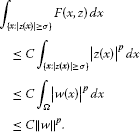(5.8)

For $x\in \mathrm{\Omega }$ with $|z\left(x\right)|⩽\sigma$, $F\left(x,z\left(x\right)\right)⩽0$. Therefore,

$\begin{array}{rcl}\mathrm{\Phi }\left(z\right)& ⩾& \frac{{\lambda }_{k+2}^{A}-{\lambda }_{k+1}^{A}}{2{\lambda }_{k+2}^{A}}{\parallel w\parallel }^{2}-{\int }_{\left\{x:|z\left(x\right)|⩽\sigma \right\}}F\left(x,z\left(x\right)\right)\phantom{\rule{0.2em}{0ex}}dx-C{\parallel w\parallel }^{p}\\ ⩾& \frac{{\lambda }_{k+2}^{A}-{\lambda }_{k+1}^{A}}{2{\lambda }_{k+2}^{A}}{\parallel w\parallel }^{2}-C{\parallel w\parallel }^{p}.\end{array}$
(5.9)

Since $p>2$, for $r>0$ small,

(5.10)

For $z=y\in E\left({\lambda }_{k+1}^{A}\right)$, it must hold that

(5.11)

Here we use a potential convention that (GS) λ has finitely many solutions and then 0 is isolated. Otherwise, one would have that as $r>0$ small, $\parallel y\parallel ⩽r$ implies $|y\left(x\right)|⩽\delta$ for all $x\in \mathrm{\Omega }$, $\mathrm{\nabla }F\left(x,y\right)\equiv 0$ for all $x\in \mathrm{\Omega }$. Thus, 0 would not be an isolated critical point of Φ and (GS) λ would have infinitely many nontrivial solutions. By (5.10) and (5.11), we verify that

Applying Proposition 2.2, we obtain

${C}_{q}\left(\mathrm{\Phi },0\right)={\delta }_{q,{\ell }_{k}}\mathbb{F}.$

(4) When $F\left(x,z\right)⩾0$ for $|z|$ small, a similar argument shows that Φ has a local linking structure at $z=0$ with respect to $E={E}_{k+1}\oplus {E}_{k+1}^{\perp }$. By Proposition 2.2, it follows that ${C}_{q}\left(\mathrm{\Phi },0\right)\cong {\delta }_{q,{\ell }_{k+1}}\mathbb{F}$. □

Finally, we prove the theorems.

Proof of Theorem 1.1 It follows from (${F}_{5}$) that $F\left(x,z\right)⩾0$ for $|z|>0$ small. By Theorem 3.4 for the part $\lambda \in \left({\lambda }_{k+1}^{A}-\delta ,{\lambda }_{k+1}^{A}\right)$, (GS) λ has a nontrivial solution ${z}^{\lambda }$ satisfying

${C}_{{\ell }_{k+1}+1}\left(\mathrm{\Phi },{z}^{\lambda }\right)\ncong 0.$
(5.12)

By Theorem 4.2(1), (GS) λ has two nontrivial solutions ${z}_{\lambda }^{i}$ ($i=1,2$) with their Morse indices satisfying

${\ell }_{k}⩽m\left({z}_{\lambda }^{i}\right)⩽m\left({z}_{\lambda }^{i}\right)+n\left({z}_{\lambda }^{i}\right)⩽{\ell }_{k+1},\phantom{\rule{1em}{0ex}}i=1,2.$

From Proposition 2.1(2), we have that

${C}_{q}\left(\mathrm{\Phi },{z}_{\lambda }^{i}\right)\cong 0,\phantom{\rule{1em}{0ex}}q\notin \left[{\ell }_{k},{\ell }_{k+1}\right],i=1,2.$
(5.13)

From (5.12) and (5.13), we see that ${z}^{\lambda }\ne {z}_{\lambda }^{i}$ ($i=1,2$). The proof is complete. □

Proof of Theorem 1.2 With the same argument as above, it follows from Theorem 4.2(2) and Theorem 3.4 for the part $\lambda \in \left({\lambda }_{k+1}^{A},{\lambda }_{k+1}^{A}+\delta \right)$. We omit the details. □

Proof of Theorem 1.3 By Theorem 3.4 for the part $\lambda \in \left({\lambda }_{k+1}^{A}-\delta ,{\lambda }_{k+1}^{A}\right]$, (GS) λ has a solution ${z}^{\lambda }$ with its energy $\mathrm{\Phi }\left({z}^{\lambda }\right)⩾\alpha >0$ and

${C}_{{\ell }_{k+1}+1}\left(\mathrm{\Phi },{z}^{\lambda }\right)\ncong 0.$
(5.14)

By Lemma 5.1 and Lemma 5.2(3), we have that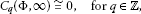(5.15)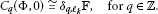(5.16)

Assume that (GS) λ has only two solutions 0 and ${z}^{\lambda }$. Choose $a,b\in \mathbb{R}$ such that $a<0. Then by the deformation and excision properties of singular homology (see ), we have

$\left\{\begin{array}{c}{C}_{q}\left(\mathrm{\Phi },\mathrm{\infty }\right)\cong {H}_{q}\left(E,{\mathrm{\Phi }}^{a}\right);\hfill \\ {C}_{q}\left(\mathrm{\Phi },0\right)\cong {H}_{q}\left({\mathrm{\Phi }}^{b},{\mathrm{\Phi }}^{a}\right);\hfill \\ {C}_{q}\left(\mathrm{\Phi },{z}^{\lambda }\right)\cong {H}_{q}\left(E,{\mathrm{\Phi }}^{b}\right).\hfill \end{array}$
(5.17)

By (5.17), the long exact sequences for the topological triple $\left(E,{\mathrm{\Phi }}^{b},{\mathrm{\Phi }}^{a}\right)$ read as

$\cdots \to {C}_{q+1}\left(\mathrm{\Phi },\mathrm{\infty }\right)\to {C}_{q+1}\left(\mathrm{\Phi },{z}^{\lambda }\right)\to {C}_{q}\left(\mathrm{\Phi },0\right)\to {C}_{q}\left(\mathrm{\Phi },\mathrm{\infty }\right)\to \cdots .$
(5.18)

We deduce by (5.15) and (5.18) that

(5.19)

Take $q={\ell }_{k+1}$ in (5.19), then

${C}_{{\ell }_{k+1}+1}\left(\mathrm{\Phi },{z}^{\lambda }\right)\cong {C}_{{\ell }_{k+1}}\left(\mathrm{\Phi },0\right)\cong 0$

which contradicts (5.14). The proof is complete. □

We finally remark that Theorem 1.1 is valid for $\lambda \in \left({\lambda }_{1}^{A}-\delta ,{\lambda }_{1}^{A}\right)$, from which one sees that $z=0$ is a local minimizer of Φ.

## References

1. Rabinowitz PH CBMS Reg. Conf. Ser. Math. 65. In Minimax Methods in Critical Point Theory with Applications to Differential Equations. Am. Math. Soc., Providence; 1986.

2. Chang KC: An extension of the Hess-Kato theorem to elliptic systems and its applications to multiple solutions problems. Acta Math. Sin. 1999, 15: 439-454. 10.1007/s10114-999-0078-0

3. Chang KC: Principal eigenvalue for weight in elliptic systems. Nonlinear Anal. 2001, 46: 419-433. 10.1016/S0362-546X(00)00140-1

4. Ambrosetti A, Rabinowitz PH: Dual variational methods in critical point theory and applications. J. Funct. Anal. 1973, 14: 349-381. 10.1016/0022-1236(73)90051-7

5. Wang ZQ: On a superlinear elliptic equation. Ann. Inst. Henri Poincaré, Anal. Non Linéaire 1991, 8: 43-57.

6. Rabinowitz PH: Some critical point theorems and applications to semilinear elliptic partial differential equations. Ann. Sc. Norm. Super. Pisa, Cl. Sci. 1978, 5: 215-223.

7. Li SJ, Liu JQ: Some existence theorems on multiple critical points and their applications. Kexue Tongbao 1984, 17: 1025-1027.

8. Li SJ, Willem M: Applications of local linking to critical point theory. J. Math. Anal. Appl. 1995, 189: 6-32. 10.1006/jmaa.1995.1002

9. Rabinowitz PH, Su JB, Wang ZQ: Multiple solutions of superlinear elliptic equations. Rend. Lincei Mat. Appl. 2007, 18: 97-108.

10. Li XL, Su JB, Tian RS: Multiple periodic solutions of the second order Hamiltonian systems with superlinear terms. J. Math. Anal. Appl. 2012, 385: 1-11. 10.1016/j.jmaa.2011.06.061

11. Mawhin J, Willem M: Critical Point Theory and Hamiltonian Systems. 1st edition. Springer, Berlin; 1989.

12. Chang KC: Infinite Dimensional Morse Theory and Multiple Solutions Problems. 1st edition. Birkhäuser, Boston; 1993.

13. Bartsch T, Li SJ: Critical point theory for asymptotically quadratic functionals and applications to problems with resonance. Nonlinear Anal. 1997, 28: 419-441. 10.1016/0362-546X(95)00167-T

14. Gromoll D, Meyer M: On differential functions with isolated point. Topology 1969, 8: 361-369. 10.1016/0040-9383(69)90022-6

15. Liu JQ: A Morse index for a saddle point. Syst. Sci. Math. Sci. 1989, 2: 32-39.

16. Su JB: Multiplicity results for asymptotically linear elliptic problems at resonance. J. Math. Anal. Appl. 2003, 278: 397-408. 10.1016/S0022-247X(02)00707-2

17. Gilbarg D, Trudinger NS: Elliptic Partial Differential Equations of the Second Order. 2nd edition. Springer, Berlin; 1983.

## Acknowledgements

The authors are grateful to the anonymous referee for his/her valuable suggestions. The second author was supported by NSFC11271264, NSFC11171204, KZ201010028027 and PHR201106118.

## Author information

Authors

### Corresponding author

Correspondence to Anran Li.

### Competing interests

The authors declare that they have no competing interests.

### Authors’ contributions

The authors declare that the study was realized in collaboration with the same responsibility. All authors read and approved the final manuscript.

## Rights and permissions

Reprints and Permissions

Li, A., Su, J. Superlinear gradient system with a parameter. Bound Value Probl 2012, 110 (2012). https://doi.org/10.1186/1687-2770-2012-110

• Accepted:

• Published:

• DOI: https://doi.org/10.1186/1687-2770-2012-110

### Keywords